Back to Adaptive Vision Library Lite website

You are here: Start » Image Local Transforms » GradientMagnitudeImage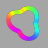Measures the strength of gradient at each pixel location with Sobel or Prewitt operator.

### Syntax

void avl::GradientMagnitudeImage
(
const avl::Image& inImage,
atl::Optional<const avl::Region&> inRoi,
avl::MagnitudeMeasure::Type inMeasure,
const int inScale,
avl::Image& outValueImage
)


### Parameters

Name Type Range Default Description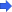inImage const Image& Input imageinRoi Optional<const Region&> NIL Range of output pixels to be computedinMeasure MagnitudeMeasure::Type Hypot Defines how the gradient magnitude is computedinScale const int 1 - 16 1 Scales the resulting gradient magnitudes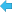outValueImage Image& Gradient magnitudes of the image

### Description

The operation computes the magnitude of the intensity change at each pixel of the inImage. Firstly the selected inOperator is used to obtain two-dimensional gradient vector at each pixel. Then the magnitudes of the vectors are estimated using the inMeasure method.

Specified by inMeasure method computes magnitude (A) from horizontal gradient component (x) and vertical gradient component (y) using one of following formulas:
$A_{Horizontal}=|x|$ $A_{Vertical}=|y|$ $A_{Average}=\frac{|x|+|y|}{2}$ $A_{Sum}=|x|+|y|$ $A_{Maximum}=Max(|x|,|y|)$ $A_{Hypot}=\sqrt{x^{2}+y^{2} }$

The magnitudes are multiplied by inScale factor and saturated if they exceed the greatest value of their type.

### Examples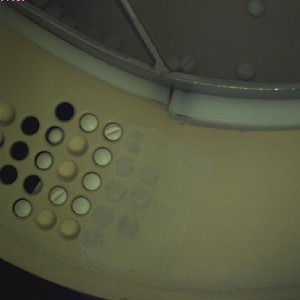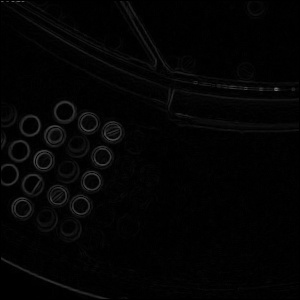GradientMagnitudeImage performed on the sample image with inOperator = Sobel, inMeasure = Hypot.

### Hardware Acceleration

This operation is optimized for SSSE3 technology for pixels of type: UINT8.

This operation is optimized for AVX2 technology for pixels of type: UINT8.

This operation is optimized for NEON technology for pixels of type: UINT8.

This operation supports automatic parallelization for multicore and multiprocessor systems.

### Errors

List of possible exceptions:

Error type Description
DomainError Region exceeds an input image in GradientMagnitudeImage.